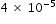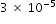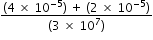# MAFS.8.EE.1.4Archived StandardExport Print
Perform operations with numbers expressed in scientific notation, including problems where both decimal and scientific notation are used. Use scientific notation and choose units of appropriate size for measurements of very large or very small quantities (e.g., use millimeters per year for seafloor spreading). Interpret scientific notation that has been generated by technology.
General Information
Subject Area: Mathematics
Domain-Subdomain: Expressions & Equations
Cluster: Level 2: Basic Application of Skills & Concepts
Cluster: Work with radicals and integer exponents. (Major Cluster) -

Clusters should not be sorted from Major to Supporting and then taught in that order. To do so would strip the coherence of the mathematical ideas and miss the opportunity to enhance the major work of the grade with the supporting clusters.

Date of Last Rating: 02/14
Status: State Board Approved - Archived
Assessed: Yes
Test Item Specifications

• Assessment Limits :

N/A

• Calculator :

No

• Context :

Allowable

Sample Test Items (2)
• Test Item #: Sample Item 1
• Question:

What is the sum ofand?

• Difficulty: N/A
• Type: EE: Equation Editor

• Test Item #: Sample Item 2
• Question:

An expression is shown.Which expression is equivalent?

• Difficulty: N/A
• Type: MC: Multiple Choice

## Related Courses

This benchmark is part of these courses.
1205050: M/J Accelerated Mathematics Grade 7 (Specifically in versions: 2014 - 2015, 2015 - 2020, 2020 - 2022, 2022 and beyond (current))
1205070: M/J Grade 8 Pre-Algebra (Specifically in versions: 2014 - 2015, 2015 - 2022, 2022 and beyond (current))
1204000: M/J Foundational Skills in Mathematics 6-8 (Specifically in versions: 2014 - 2015, 2015 - 2022, 2022 and beyond (current))
1200410: Mathematics for College Success (Specifically in versions: 2014 - 2015, 2015 - 2022 (course terminated))
1200700: Mathematics for College Algebra (Specifically in versions: 2014 - 2015, 2015 - 2022 (course terminated))
7812030: Access M/J Grade 8 Pre-Algebra (Specifically in versions: 2014 - 2015, 2015 - 2018, 2018 - 2019, 2019 - 2022, 2022 and beyond (current))

## Related Access Points

Alternate version of this benchmark for students with significant cognitive disabilities.

## Related Resources

Vetted resources educators can use to teach the concepts and skills in this benchmark.

## Formative Assessments

Sums and Differences in Scientific Notation:

Students are asked to add and subtract numbers given in scientific notation in real-world contexts.

Type: Formative Assessment

Scientific Multiplication and Division:

Students are asked to multiply and divide numbers given in scientific notation in real-world contexts.

Type: Formative Assessment

Mixed Form Operations:

Students are given word problems with numbers in both standard and scientific notation and asked to solve problems using various operations.

Type: Formative Assessment

Estimating Length Using Scientific Notation:

This lesson unit is intended to help you assess how well students are able to:

• Estimate lengths of everyday objects.
• Convert between decimal and scientific notation.
• Make comparisons of the size of numbers expressed in both decimal and scientific notation.

Type: Formative Assessment

Scientific Calculator Display:

Students are given examples of calculator displays and asked to convert the notation in the display to both scientific notation and standard form.

Type: Formative Assessment

## Lesson Plans

Which Map Should We Use?:

Students will create a working model of the solar system to scale. They will incorporate QR codes to present information on solar system objects, as well as compare the geocentric and heliocentric models of the solar system.

Type: Lesson Plan

How Many Smoots Does It Take to Reach the Moon? :

In this discovery oriented lesson, students will explore the use of non-standard units of measurement. They will convert linear measurements within the metric system and also convert measurements given in astronomical units (AU) into more familiar units, specifically meters and kilometers. The unit conversions will be completed with measurements that are expressed in scientific notation. Students will recall their prior knowledge of how to add and subtract numbers given in scientific notation. They will also use their knowledge of exponent rules to determine an efficient method for multiplying and dividing numbers expressed in scientific notation.

Type: Lesson Plan

Estimating Length Using Scientific Notation:

This lesson unit is intended to help you assess how well students are able to estimate lengths of everyday objects, convert between decimal and scientific notation and make comparisons of the size of numbers expressed in both decimal and scientific notation.

Type: Lesson Plan

Discovering Kepler's Law for the Periods of Planets:

Students listen to a video that describes Kepler's determination that planetary orbits are elliptical and then will use data for the solar distance and periods of several of the planets in the solar system, then investigate several hypotheses to determine which is supported by the data.

Type: Lesson Plan

Pennies to Heaven:

The goal of this task is to give students a context to investigate large numbers and measurements. Students need to fluently convert units with very large numbers in order to successfully complete this task. The total number of pennies minted either in a single year or for the last century is phenomenally large and difficult to grasp. One way to assess how large this number is would be to consider how far all of these pennies would reach if we were able to stack them one on top of another: this is another phenomenally large number but just how large may well come as a surprise.

Ants versus humans:

This task requires students to work with very large and small values expressed both in scientific notation and in decimal notation (standard form). In addition, students need to convert units of mass. The solution below converts the mass of humans into grams; however, we could just as easily converted the mass of ants into kilograms. Students are unable to go directly to a calculator without taking into account all of the considerations mentioned above. Even after converting units and decimals to scientific notation, students should be encouraged to use the structure of scientific notation to regroup the products by extending the properties of operations and then use the properties of exponents to more fluently perform the calculations involved rather than rely heavily on a calculator.

Giantburgers:

The student is asked to perform operations with numbers expressed in scientific notation to decide whether 7% of Americans really do eat at Giantburger every day.

## Student Center Activity

Students can practice answering mathematics questions on a variety of topics. With an account, students can save their work and send it to their teacher when complete.

Type: Student Center Activity

## Tutorials

Multiplying in Scientific Notation:

This video demonstrates multiplying in scientific notation.

Type: Tutorial

Calculating Red Blood Cells in the Body Using Scientific Notation:

This example demonstrates mathematical operations with scientific notation used to solve a word problem.

Type: Tutorial

U.S. National Debt (Scientific Notation Word Problem):

This video demonstrates a scientific notation word problem involving division.

Type: Tutorial

Simplifying an Expression into Scientific Notation:

This is an example showing how to simplify an expression into scientific notation.

Type: Tutorial

Scientific Notation:

Scientific notation is used to conveniently write numbers that require many digits in their representations. How to convert between standard and scientific notation is explained in this tutorial.

Type: Tutorial

## MFAS Formative Assessments

Mixed Form Operations:

Students are given word problems with numbers in both standard and scientific notation and asked to solve problems using various operations.

Scientific Calculator Display:

Students are given examples of calculator displays and asked to convert the notation in the display to both scientific notation and standard form.

Scientific Multiplication and Division:

Students are asked to multiply and divide numbers given in scientific notation in real-world contexts.

Sums and Differences in Scientific Notation:

Students are asked to add and subtract numbers given in scientific notation in real-world contexts.

## Student Resources

Vetted resources students can use to learn the concepts and skills in this benchmark.

Pennies to Heaven:

The goal of this task is to give students a context to investigate large numbers and measurements. Students need to fluently convert units with very large numbers in order to successfully complete this task. The total number of pennies minted either in a single year or for the last century is phenomenally large and difficult to grasp. One way to assess how large this number is would be to consider how far all of these pennies would reach if we were able to stack them one on top of another: this is another phenomenally large number but just how large may well come as a surprise.

Giantburgers:

The student is asked to perform operations with numbers expressed in scientific notation to decide whether 7% of Americans really do eat at Giantburger every day.

## Student Center Activity

Students can practice answering mathematics questions on a variety of topics. With an account, students can save their work and send it to their teacher when complete.

Type: Student Center Activity

## Tutorials

Multiplying in Scientific Notation:

This video demonstrates multiplying in scientific notation.

Type: Tutorial

Calculating Red Blood Cells in the Body Using Scientific Notation:

This example demonstrates mathematical operations with scientific notation used to solve a word problem.

Type: Tutorial

U.S. National Debt (Scientific Notation Word Problem):

This video demonstrates a scientific notation word problem involving division.

Type: Tutorial

Simplifying an Expression into Scientific Notation:

This is an example showing how to simplify an expression into scientific notation.

Type: Tutorial

Scientific Notation:

Scientific notation is used to conveniently write numbers that require many digits in their representations. How to convert between standard and scientific notation is explained in this tutorial.

Type: Tutorial

## Parent Resources

Vetted resources caregivers can use to help students learn the concepts and skills in this benchmark.

Pennies to Heaven:

The goal of this task is to give students a context to investigate large numbers and measurements. Students need to fluently convert units with very large numbers in order to successfully complete this task. The total number of pennies minted either in a single year or for the last century is phenomenally large and difficult to grasp. One way to assess how large this number is would be to consider how far all of these pennies would reach if we were able to stack them one on top of another: this is another phenomenally large number but just how large may well come as a surprise.